# Mean State

Period Mean (original grids) [K]
Model Period Mean (intersection) [K]
Model Period Mean (complement) [K]
Benchmark Period Mean (intersection) [K]
Benchmark Period Mean (complement) [K]
Bias [K]
RMSE [K]
Phase Shift [months]
Bias Score 
RMSE Score 
Seasonal Cycle Score 
Spatial Distribution Score 
Interannual Variability Score 
Overall Score 
Benchmark [-] 299.
CLM4 [-] 298. 298. 301. 298. 300. -0.366 1.05 0.353 0.46 0.76 0.96 0.94 0.93 0.80
CLM4.5 [-] 298. 298. 300. 298. 300. -0.147 0.947 0.322 0.50 0.78 0.97 0.96 0.94 0.82
CLM5 [-] 299. 299. 301. 298. 300. 0.167 0.738 0.323 0.62 0.79 0.96 0.98 0.96 0.85
Period Mean (original grids) [K]
Model Period Mean (intersection) [K]
Model Period Mean (complement) [K]
Benchmark Period Mean (intersection) [K]
Benchmark Period Mean (complement) [K]
Bias [K]
RMSE [K]
Phase Shift [months]
Bias Score 
RMSE Score 
Seasonal Cycle Score 
Spatial Distribution Score 
Interannual Variability Score 
Overall Score 
Benchmark [-] 300.
CLM4 [-] 300. 300. 301. 300. 300. 0.0789 0.911 0.228 0.76 0.79 0.98 1.0 0.95 0.88
CLM4.5 [-] 300. 301. 301. 300. 300. 0.316 0.883 0.221 0.74 0.82 0.98 1.0 0.96 0.89
CLM5 [-] 301. 301. 301. 300. 300. 0.521 0.852 0.195 0.73 0.85 0.98 1.0 0.97 0.90
Period Mean (original grids) [K]
Model Period Mean (intersection) [K]
Model Period Mean (complement) [K]
Benchmark Period Mean (intersection) [K]
Benchmark Period Mean (complement) [K]
Bias [K]
RMSE [K]
Phase Shift [months]
Bias Score 
RMSE Score 
Seasonal Cycle Score 
Spatial Distribution Score 
Interannual Variability Score 
Overall Score 
Benchmark [-] 295.
CLM4 [-] 295. 295. 296. 295. 297. 0.0992 0.933 0.410 0.69 0.83 0.95 1.0 0.92 0.87
CLM4.5 [-] 295. 295. 296. 295. 297. 0.336 0.850 0.334 0.72 0.85 0.96 1.0 0.93 0.89
CLM5 [-] 296. 295. 297. 295. 297. 0.445 0.865 0.404 0.71 0.87 0.95 1.0 0.95 0.89
Period Mean (original grids) [K]
Model Period Mean (intersection) [K]
Model Period Mean (complement) [K]
Benchmark Period Mean (intersection) [K]
Benchmark Period Mean (complement) [K]
Bias [K]
RMSE [K]
Phase Shift [months]
Bias Score 
RMSE Score 
Seasonal Cycle Score 
Spatial Distribution Score 
Interannual Variability Score 
Overall Score 
Benchmark [-] 262.
CLM4 [-] 258. 258. 262. 262. 266. -2.90 3.47 0.0203 0.83 0.89 1.0 1.0 0.92 0.92
CLM4.5 [-] 259. 259. 262. 262. 266. -2.58 3.20 0.0161 0.85 0.90 1.0 1.0 0.91 0.92
CLM5 [-] 260. 260. 263. 262. 266. -1.00 1.60 0.0159 0.92 0.94 1.0 1.0 0.92 0.95
Period Mean (original grids) [K]
Model Period Mean (intersection) [K]
Model Period Mean (complement) [K]
Benchmark Period Mean (intersection) [K]
Benchmark Period Mean (complement) [K]
Bias [K]
RMSE [K]
Phase Shift [months]
Bias Score 
RMSE Score 
Seasonal Cycle Score 
Spatial Distribution Score 
Interannual Variability Score 
Overall Score 
Benchmark [-] 299.
CLM4 [-] 298. 298. 299. 299. 299. -0.555 1.01 0.773 0.33 0.60 0.89 0.97 0.85 0.71
CLM4.5 [-] 299. 298. 299. 299. 299. -0.452 0.944 0.717 0.35 0.62 0.90 0.98 0.86 0.72
CLM5 [-] 299. 299. 300. 299. 299. -0.0330 0.793 0.616 0.42 0.68 0.91 1.0 0.88 0.76
Period Mean (original grids) [K]
Model Period Mean (intersection) [K]
Model Period Mean (complement) [K]
Benchmark Period Mean (intersection) [K]
Benchmark Period Mean (complement) [K]
Bias [K]
RMSE [K]
Phase Shift [months]
Bias Score 
RMSE Score 
Seasonal Cycle Score 
Spatial Distribution Score 
Interannual Variability Score 
Overall Score 
Benchmark [-] 271.
CLM4 [-] 269. 269. 273. 271. 274. -2.43 2.85 0.00954 0.84 0.91 1.0 1.0 0.97 0.94
CLM4.5 [-] 269. 269. 273. 271. 274. -2.28 2.73 0.00954 0.85 0.91 1.0 1.0 0.97 0.94
CLM5 [-] 271. 270. 274. 271. 274. -0.737 1.06 0.00890 0.94 0.96 1.0 1.0 0.98 0.97
Period Mean (original grids) [K]
Model Period Mean (intersection) [K]
Model Period Mean (complement) [K]
Benchmark Period Mean (intersection) [K]
Benchmark Period Mean (complement) [K]
Bias [K]
RMSE [K]
Phase Shift [months]
Bias Score 
RMSE Score 
Seasonal Cycle Score 
Spatial Distribution Score 
Interannual Variability Score 
Overall Score 
Benchmark [-] 280.
CLM4 [-] 279. 279. 287. 280. 285. -0.945 1.80 0.0264 0.86 0.91 1.0 1.0 0.94 0.94
CLM4.5 [-] 280. 279. 287. 280. 285. -0.792 1.70 0.0264 0.87 0.92 1.0 1.0 0.94 0.94
CLM5 [-] 280. 280. 287. 280. 285. -0.0730 1.17 0.0271 0.90 0.95 1.0 1.0 0.96 0.96
Period Mean (original grids) [K]
Model Period Mean (intersection) [K]
Model Period Mean (complement) [K]
Benchmark Period Mean (intersection) [K]
Benchmark Period Mean (complement) [K]
Bias [K]
RMSE [K]
Phase Shift [months]
Bias Score 
RMSE Score 
Seasonal Cycle Score 
Spatial Distribution Score 
Interannual Variability Score 
Overall Score 
Benchmark [-] 297.
CLM4 [-] 296. 296. 300. 297. 300. -0.323 1.31 0.180 0.74 0.85 0.98 0.99 0.91 0.89
CLM4.5 [-] 297. 297. 300. 297. 300. -0.121 1.24 0.181 0.75 0.86 0.98 0.99 0.92 0.89
CLM5 [-] 297. 297. 300. 297. 300. 0.195 1.12 0.170 0.75 0.89 0.98 1.0 0.94 0.91
Period Mean (original grids) [K]
Model Period Mean (intersection) [K]
Model Period Mean (complement) [K]
Benchmark Period Mean (intersection) [K]
Benchmark Period Mean (complement) [K]
Bias [K]
RMSE [K]
Phase Shift [months]
Bias Score 
RMSE Score 
Seasonal Cycle Score 
Spatial Distribution Score 
Interannual Variability Score 
Overall Score 
Benchmark [-] 264.
CLM4 [-] 261. 261. 262. 264. 264. -3.09 3.70 0.00943 0.82 0.88 1.0 1.0 0.96 0.92
CLM4.5 [-] 261. 261. 262. 264. 264. -2.91 3.46 0.0496 0.83 0.89 1.0 1.0 0.96 0.93
CLM5 [-] 263. 263. 264. 264. 264. -1.09 1.43 0.0193 0.93 0.95 1.0 1.0 0.97 0.97
Period Mean (original grids) [K]
Model Period Mean (intersection) [K]
Model Period Mean (complement) [K]
Benchmark Period Mean (intersection) [K]
Benchmark Period Mean (complement) [K]
Bias [K]
RMSE [K]
Phase Shift [months]
Bias Score 
RMSE Score 
Seasonal Cycle Score 
Spatial Distribution Score 
Interannual Variability Score 
Overall Score 
Benchmark [-] 295.
CLM4 [-] 295. 295. 293. 295. 294. 0.0117 0.641 0.110 0.90 0.91 0.99 1.0 0.93 0.94
CLM4.5 [-] 295. 295. 293. 295. 294. 0.00772 0.650 0.111 0.90 0.91 0.99 1.0 0.93 0.94
CLM5 [-] 295. 295. 293. 295. 294. 0.323 0.624 0.0892 0.89 0.93 0.99 1.0 0.95 0.95
Period Mean (original grids) [K]
Model Period Mean (intersection) [K]
Model Period Mean (complement) [K]
Benchmark Period Mean (intersection) [K]
Benchmark Period Mean (complement) [K]
Bias [K]
RMSE [K]
Phase Shift [months]
Bias Score 
RMSE Score 
Seasonal Cycle Score 
Spatial Distribution Score 
Interannual Variability Score 
Overall Score 
Benchmark [-] 282.
CLM4 [-] 281. 281. 283. 282. 286. -1.00 1.69 0.124 0.84 0.87 0.99 0.98 0.91 0.91
CLM4.5 [-] 281. 281. 283. 282. 286. -1.02 1.67 0.128 0.83 0.87 0.99 0.99 0.91 0.91
CLM5 [-] 281. 281. 284. 282. 286. -0.172 0.983 0.147 0.89 0.92 0.99 1.0 0.95 0.95
Period Mean (original grids) [K]
Model Period Mean (intersection) [K]
Model Period Mean (complement) [K]
Benchmark Period Mean (intersection) [K]
Benchmark Period Mean (complement) [K]
Bias [K]
RMSE [K]
Phase Shift [months]
Bias Score 
RMSE Score 
Seasonal Cycle Score 
Spatial Distribution Score 
Interannual Variability Score 
Overall Score 
Benchmark [-] 287.
CLM4 [-] 281. 286. 243. 287. 292. -0.921 1.65 0.173 0.77 0.86 0.98 0.99 0.93 0.90
CLM4.5 [-] 281. 286. 243. 287. 292. -0.792 1.58 0.164 0.78 0.86 0.98 0.99 0.94 0.90
CLM5 [-] 282. 286. 244. 287. 292. -0.107 1.02 0.157 0.82 0.90 0.98 1.0 0.95 0.93
Period Mean (original grids) [K]
Model Period Mean (intersection) [K]
Model Period Mean (complement) [K]
Benchmark Period Mean (intersection) [K]
Benchmark Period Mean (complement) [K]
Bias [K]
RMSE [K]
Phase Shift [months]
Bias Score 
RMSE Score 
Seasonal Cycle Score 
Spatial Distribution Score 
Interannual Variability Score 
Overall Score 
Benchmark [-] 296.
CLM4 [-] 296. 296. 296. 296. 297. -0.125 1.01 0.152 0.88 0.92 0.99 1.0 0.96 0.94
CLM4.5 [-] 296. 296. 296. 296. 297. -0.0557 0.978 0.149 0.89 0.92 0.99 1.0 0.96 0.95
CLM5 [-] 296. 296. 297. 296. 297. 0.240 0.867 0.138 0.89 0.94 0.99 1.0 0.96 0.95
Period Mean (original grids) [K]
Model Period Mean (intersection) [K]
Model Period Mean (complement) [K]
Benchmark Period Mean (intersection) [K]
Benchmark Period Mean (complement) [K]
Bias [K]
RMSE [K]
Phase Shift [months]
Bias Score 
RMSE Score 
Seasonal Cycle Score 
Spatial Distribution Score 
Interannual Variability Score 
Overall Score 
Benchmark [-] 265.
CLM4 [-] 262. 262. 260. 265. 266. -2.77 3.36 0.0230 0.81 0.87 1.0 0.99 0.94 0.91
CLM4.5 [-] 262. 262. 260. 265. 266. -2.60 3.21 0.0179 0.82 0.87 1.0 0.99 0.94 0.92
CLM5 [-] 264. 264. 261. 265. 266. -0.989 1.43 0.0106 0.92 0.93 1.0 1.0 0.94 0.96
Period Mean (original grids) [K]
Model Period Mean (intersection) [K]
Model Period Mean (complement) [K]
Benchmark Period Mean (intersection) [K]
Benchmark Period Mean (complement) [K]
Bias [K]
RMSE [K]
Phase Shift [months]
Bias Score 
RMSE Score 
Seasonal Cycle Score 
Spatial Distribution Score 
Interannual Variability Score 
Overall Score 
Benchmark [-] 296.
CLM4 [-] 295. 295. 298. 296. 298. -0.0641 1.27 0.269 0.66 0.83 0.98 1.0 0.91 0.87
CLM4.5 [-] 295. 295. 298. 296. 298. -0.107 1.25 0.293 0.67 0.81 0.97 1.0 0.91 0.86
CLM5 [-] 295. 295. 298. 296. 298. 0.206 1.20 0.263 0.67 0.85 0.98 1.0 0.93 0.88
Period Mean (original grids) [K]
Model Period Mean (intersection) [K]
Model Period Mean (complement) [K]
Benchmark Period Mean (intersection) [K]
Benchmark Period Mean (complement) [K]
Bias [K]
RMSE [K]
Phase Shift [months]
Bias Score 
RMSE Score 
Seasonal Cycle Score 
Spatial Distribution Score 
Interannual Variability Score 
Overall Score 
Benchmark [-] 286.
CLM4 [-] 285. 285. 293. 286. 292. -1.07 1.41 0.0428 0.88 0.91 1.0 1.0 0.95 0.94
CLM4.5 [-] 285. 285. 293. 286. 292. -0.971 1.35 0.0433 0.89 0.91 1.0 1.0 0.96 0.94
CLM5 [-] 286. 286. 294. 286. 292. -0.0907 0.670 0.0345 0.95 0.95 1.0 1.0 0.97 0.97
Period Mean (original grids) [K]
Model Period Mean (intersection) [K]
Model Period Mean (complement) [K]
Benchmark Period Mean (intersection) [K]
Benchmark Period Mean (complement) [K]
Bias [K]
RMSE [K]
Phase Shift [months]
Bias Score 
RMSE Score 
Seasonal Cycle Score 
Spatial Distribution Score 
Interannual Variability Score 
Overall Score 
Benchmark [-] 275.
CLM4 [-] 272. 272. 276. 274. 278. -2.21 2.59 0.0546 0.81 0.90 1.0 0.97 0.96 0.92
CLM4.5 [-] 272. 272. 276. 274. 278. -2.08 2.48 0.0553 0.82 0.89 1.0 0.97 0.97 0.93
CLM5 [-] 274. 274. 277. 274. 278. -0.630 1.01 0.0424 0.92 0.95 1.0 1.0 0.97 0.96
Period Mean (original grids) [K]
Model Period Mean (intersection) [K]
Model Period Mean (complement) [K]
Benchmark Period Mean (intersection) [K]
Benchmark Period Mean (complement) [K]
Bias [K]
RMSE [K]
Phase Shift [months]
Bias Score 
RMSE Score 
Seasonal Cycle Score 
Spatial Distribution Score 
Interannual Variability Score 
Overall Score 
Benchmark [-] 284.
CLM4 [-] 282. 283. 286. 284. 286. -0.886 1.75 0.0307 0.85 0.89 1.0 0.99 0.93 0.92
CLM4.5 [-] 282. 283. 286. 284. 286. -0.805 1.75 0.0295 0.85 0.88 1.0 1.0 0.93 0.92
CLM5 [-] 283. 283. 287. 284. 286. -0.0645 1.18 0.0249 0.88 0.92 1.0 1.0 0.97 0.95
Period Mean (original grids) [K]
Model Period Mean (intersection) [K]
Model Period Mean (complement) [K]
Benchmark Period Mean (intersection) [K]
Benchmark Period Mean (complement) [K]
Bias [K]
RMSE [K]
Phase Shift [months]
Bias Score 
RMSE Score 
Seasonal Cycle Score 
Spatial Distribution Score 
Interannual Variability Score 
Overall Score 
Benchmark [-] 299.
CLM4 [-] 298. 298. 300. 299. 299. -0.539 0.965 0.523 0.53 0.72 0.93 0.98 0.90 0.80
CLM4.5 [-] 299. 299. 300. 299. 299. -0.364 0.863 0.491 0.60 0.73 0.93 0.98 0.91 0.81
CLM5 [-] 299. 299. 300. 299. 299. -0.0597 0.717 0.460 0.65 0.76 0.94 1.0 0.94 0.84
Period Mean (original grids) [K]
Model Period Mean (intersection) [K]
Model Period Mean (complement) [K]
Benchmark Period Mean (intersection) [K]
Benchmark Period Mean (complement) [K]
Bias [K]
RMSE [K]
Phase Shift [months]
Bias Score 
RMSE Score 
Seasonal Cycle Score 
Spatial Distribution Score 
Interannual Variability Score 
Overall Score 
Benchmark [-] 296.
CLM4 [-] 296. 296. 296. 296. 293. -0.282 0.892 0.258 0.74 0.82 0.97 0.99 0.90 0.87
CLM4.5 [-] 296. 296. 296. 296. 293. -0.108 0.818 0.271 0.76 0.83 0.97 0.99 0.91 0.88
CLM5 [-] 296. 296. 296. 296. 293. 0.242 0.663 0.248 0.78 0.87 0.97 1.0 0.94 0.90
Period Mean (original grids) [K]
Model Period Mean (intersection) [K]
Model Period Mean (complement) [K]
Benchmark Period Mean (intersection) [K]
Benchmark Period Mean (complement) [K]
Bias [K]
RMSE [K]
Phase Shift [months]
Bias Score 
RMSE Score 
Seasonal Cycle Score 
Spatial Distribution Score 
Interannual Variability Score 
Overall Score 
Benchmark [-] 284.
CLM4 [-] 283. 283. 287. 284. 285. -0.572 1.82 0.225 0.64 0.81 0.97 0.99 0.93 0.86
CLM4.5 [-] 284. 283. 287. 284. 285. -0.321 1.73 0.219 0.65 0.82 0.97 1.0 0.93 0.86
CLM5 [-] 284. 284. 288. 284. 285. 0.0843 1.53 0.206 0.67 0.85 0.98 1.0 0.94 0.88

# Temporally integrated period mean

BENCHMARK MEAN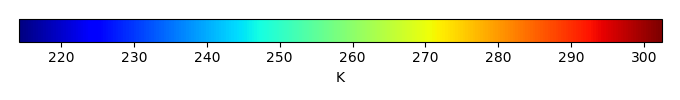MODEL MEANBIAS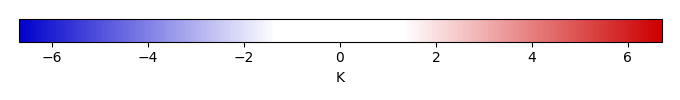BIAS SCORERMSE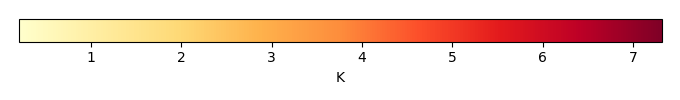RMSE SCOREBENCHMARK INTERANNUAL VARIABILITY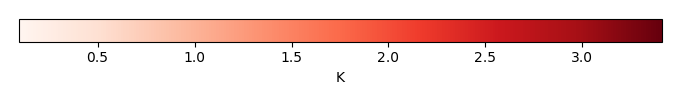MODEL INTERANNUAL VARIABILITYINTERANNUAL VARIABILITY SCOREBENCHMARK MAX MONTHMODEL MAX MONTHDIFFERENCE IN MAX MONTH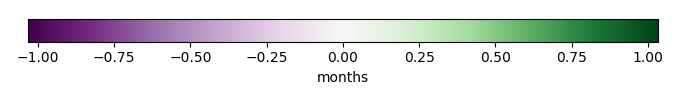SEASONAL CYCLE SCORESPATIAL TAYLOR DIAGRAMMODEL COLORS# Spatially integrated regional mean

MODEL COLORSREGIONAL MEANANNUAL CYCLEMONTHLY ANOMALYANNUAL CYCLE# All Models

BenchmarkCLM4CLM4.5CLM5# Data Information

creation_date: Fri Nov 1 11:52:30 PDT 2013

source_file: This product is generated from monthly CRU TS3.21 Mean Temperature observations

title: derived CRU high resolution (0.5x0.5) Mean Temperature

Approach: I simply read the monthly surface air temperature from original file, and separated the time series by a month and saved them by each month and each year.

Temporal resolution: monthly

General information: This product was derived from CRU v3.21 product.

Spatial resolution: 0.5x0.5 degree